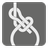Network Loops
Simple
English

A routing loop is a common problem with computer networks. Loops are virtually the same as cycles in graphs. For this mission, we’ll learn how to find these cycles in a network.

A cycle consists of a sequence of nodes starting and ending on the same node without repetition and with each consecutive node in the sequence connecting to each other in the network. The cycle length is the quantity of nodes within the cycle. The length of cycle will always be greater than 2. You should find the largest cycle in a given network, determine if we have multiple equal sized loops, then choose any one of them.

The nodes in the network are numbered and each connection is undirected. The length of the edges are not substantial, so consider them to be of equal length. Some components of a network can be disconnected.

The network is represented as a sequence of connections. Each connection is described by the two two numbers representing the connected nodes. The result should be given as a sequence of numbers which start and end with the same number. If there is no cycle, then return an empty sequence.For example this image will be described as: ((1,2),(2,3),(3,4),(4,5),(5,7),(7,6),(8,5),(8,4),(8,1),(1,5),(2,4)). And the biggest loop have 6 nodes. It can be described as [1,2,3,4,5,8,1] (or [3,4,5,8,1,2,3]).

Input: Connection information as a tuple of tuples of integers.

Output: The largest cycle, or any one of the largest cycles as a list/tuple of integers.

Example:

How it is used: Cycle detection is important for computer networking and may even be applied in the detection of deadlocks in concurrent programming.

Precondition:
all([(n2, n1) in connections for n1, n2 in connections)